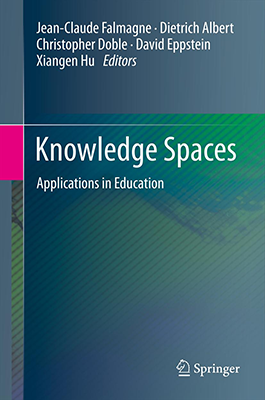So my author copies of Knowledge Spaces: Applications in Education (which I helped edit) arrived in the mail today.It's a bit of a departure for me, about computerized tutoring systems for subjects such as high school mathematics. My co-editor, Jean-Claude Falmagne, doesn't think it's appropriate to test students' knowledge of these subjects by percentages of correct answers on multiple choice exams and worksheets. Instead, for many years he's been developing a combinatorial theory of knowledge, in which a student's knowledge is represented as the set of short-answer questions that he or she can consistently answer correctly. Not all sets are possible, because of prerequisite relations between the questions. The "knowledge space" of the title is the collection of states of knowledge that are possible; mathematically, it can be represented as an antimatroid. Using this structure and some Bayesian theory, a computer can ask students a small number of questions and accurately assess not only what they know or don't know, but also what they're ready to learn next, and use that to guide the choice of the next lesson to present.

Jean-Claude's system ALEKS does exactly that. The first half of the book consists of reports of experience with ALEKS and similar systems for different groups of students and different subject areas. The second half (which is where I come in) is more mathematical, and includes several chapters on the theory of these systems. My own two chapters (heavily revised from a preprint I put on the arXiv a few years ago) describe the algorithms you need to implement to make such a system work. The first of the two chapters is primarily about an efficient computer representation of antimatroids in terms of their basic words (in learning terms, different ways to order the lessons of a subject so that, whenever a lesson is presented, students who have learned the previous lessons are ready to learn it), and algorithms that use this representation to generate all of the sets in the antimatroid (a key subroutine for the Bayesian assessment algorithm). My other chapter is about modifying knowledge spaces, for instance by restricting to a subset of the possible lessons, in order to make these spaces small enough for the algorithms run quickly.